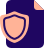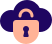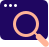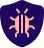Block
Are you looking to get certified in DevOps, SRE and DevSecOps?DevOps
Get Certified!SREDevSecOps
Security is KeyKubernetes
Tomorow's Platform!

# Prometheus PromQL Example Query: node exporter

## Example PromQL for Prometheus Server

``````prometheus_http_requests_total
prometheus_http_requests_total{job="prometheus",group="canary"}
prometheus_http_requests_total{environment=~"staging|testing|development",method!="GET"}
prometheus_http_requests_total{job=~".+"} # Good!
prometheus_http_requests_total{job=~".*",method="get"} # Good!
prometheus_http_requests_total[5m]
prometheus_http_requests_total{job="prometheus",group="canary"}[2h]
prometheus_http_requests_total{environment=~"staging|testing|development",method!="GET"}[60m]``````

## Prometheus PromQL Example Query: node exporter

Metrics specific to the Node Exporter are prefixed with node_ and include metrics like node_cpu_seconds_total and node_exporter_build_info.

``````Modern CPUs don't run at one constant frequency.

To save power CPUs can reduce the frequency they run at, which is quite useful for battery based devices like laptops. So while CPU metrics give you the proportion of time in each mode, one second of user time isn't always represent same amount of work as another second of user time. This can be a problem when running benchmarks.

# HELP node_cpu_frequency_max_hertz Maximum cpu thread frequency in hertz.
# TYPE node_cpu_frequency_max_hertz gauge
node_cpu_frequency_max_hertz{cpu="0"} 3.4e+09
node_cpu_frequency_max_hertz{cpu="1"} 3.4e+09

# HELP node_cpu_frequency_min_hertz Minimum cpu thread frequency in hertz.
# TYPE node_cpu_frequency_min_hertz gauge
node_cpu_frequency_min_hertz{cpu="0"} 1.6e+09
node_cpu_frequency_min_hertz{cpu="1"} 1.6e+09

# HELP node_cpu_scaling_frequency_hertz Current scaled cpu thread frequency in hertz.
# TYPE node_cpu_scaling_frequency_hertz gauge
node_cpu_scaling_frequency_hertz{cpu="0"} 2.352192e+09
node_cpu_scaling_frequency_hertz{cpu="1"} 2.243048e+09

# HELP node_cpu_scaling_frequency_max_hertz Maximum scaled cpu thread frequency in hertz.
# TYPE node_cpu_scaling_frequency_max_hertz gauge
node_cpu_scaling_frequency_max_hertz{cpu="0"} 3.4e+09
node_cpu_scaling_frequency_max_hertz{cpu="1"} 3.4e+09

# HELP node_cpu_scaling_frequency_min_hertz Minimum scaled cpu thread frequency in hertz.
# TYPE node_cpu_scaling_frequency_min_hertz gauge
node_cpu_scaling_frequency_min_hertz{cpu="0"} 1.6e+09
node_cpu_scaling_frequency_min_hertz{cpu="1"} 1.6e+09

# Linux provides scheduling metrics in /proc/schedstat, which the node exporter uses.
# The node exporter current exposes three of these:

# HELP node_schedstat_running_seconds_total Number of seconds CPU spent running a process.
# TYPE node_schedstat_running_seconds_total counter
node_schedstat_running_seconds_total{cpu="0"} 1.093032217430793e+06
node_schedstat_running_seconds_total{cpu="1"} 1.07527722232456e+06

# HELP node_schedstat_timeslices_total Number of timeslices executed by CPU.
# TYPE node_schedstat_timeslices_total counter
node_schedstat_timeslices_total{cpu="0"} 5.965185464e+09
node_schedstat_timeslices_total{cpu="1"} 5.266658269e+09

# HELP node_schedstat_waiting_seconds_total Number of seconds spent by processing waiting for this CPU.
# TYPE node_schedstat_waiting_seconds_total counter
node_schedstat_waiting_seconds_total{cpu="0"} 217918.365216207
node_schedstat_waiting_seconds_total{cpu="1"} 218559.331226331

Using the group() aggregator in PromQL

If you wanted to count the number of unique values a label has, such as say the number of values the cpu label had in node_cpu_seconds_total per instance the standard pattern is:

count without(cpu) (
count without(mode) (node_cpu_seconds_total)
)

count without(cpu) (
group without(mode) (node_cpu_seconds_total)
)

Network interface metrics from the node exporter

Along with many others, the node exporter exposes network interface metrics.

Network interface metrics have the prefix node_network_ on the node exporter's /metrics, and a device label. These are distinct from the node_netstat_ metrics which are about the kernel's network subsystem in general.

rate(node_network_transmit_errs_total[5m])
/
rate(node_network_transmit_packets_total[5m])
``````

CPU scheduling metrics from the node exporter

CPU frequency scaling metrics from the node exporter

ARP cache metrics from the node exporter

Using the group() aggregator in PromQL

Linux software RAID metrics from the node exporter

Time metric from the node exporter

Using Letsencrypt with the node exporter

Conntrack metrics from the node exporter

Kernel file descriptor metrics from the node exporter

Temperature and hardware monitoring metrics from the node exporter

Network interface metrics from the node exporter

Filesystem metrics from the node exporter

Analyse a metric by kernel version

Mapping iostat to the node exporter’s node_disk_* metrics

Using the textfile collector from a shell script

New Features in Node Exporter 0.16.0

Using group_left to calculate label proportions

Understanding Machine CPU usage

Monitoring directory sizes with the Textfile Collector